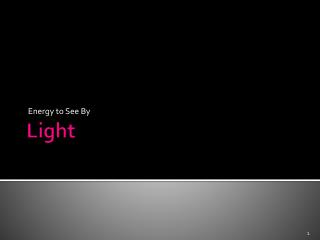DownloadDownload PresentationLight

# Light

Download Presentation## Light

- - - - - - - - - - - - - - - - - - - - - - - - - - - E N D - - - - - - - - - - - - - - - - - - - - - - - - - - -
##### Presentation Transcript

1. Energy to See By Light

2. Introduction to Light • Alien Attack Website: http://www.engineeringinteract.org/resources/alienattack.htm

3. Light Rays and Sources • Light must come from a light source. • A light source is something that produces light. • The sun and a burning candle are light sources.

4. Light Rays and Sources • Light travels in a straight line. • We use arrows to show light rays that are produced by light sources. • Black Box Experiment • What do you see in the box? Why?

5. Shadows • Shadows occur when an object blocks light from a light source. • The size of the shadow changes depending on where the object and light source are placed. • More light is blocked when the object is near the light source. This makes a bigger shadow.

6. Opaque, Transparent or Translucent • An object that does not allow light through is called opaque. • Objects or substances that allow all, or nearly all, light through them are called transparent. • Objects that allow some light to pass through them are called translucent.

7. Opaque, Transparent or Translucent A translucent material transmits light, but unlike a transparent material, it also scatters the light so those objects behind the material cannot be seen clearly.

8. What Is Light? • Light is a type of energy that travels as a wave. • Light does not require matter to travel through. • Light travels in the form of electromagnetic waves. Electromagnetic wave: a wave that consists of electric and magnetic fields that vibrate at right angles to each other

9. What Is Light? • Some properties of light can be explained only by using a particle model of light. • Light is thought of as a stream of particles called photons.

10. The Speed of Light • In the near vacuum of space, the speed of light is about 300,000,000 m/s or 300,000 km/s. • It take about 8.3 minutes for light to travel from the sun to Earth, because the Earth is 150,000,000km from the sun.

11. The Speed of Light • The distance from Earth to the moon is 384,000 km. Calculate the time it takes for light to travel that distance. • Write the equation for speed: speed = distance/time • Rearrange the equation by multiplying by time and dividing by speed time = distance/speed • Replace distance and speed with the values given: time = 384,000km/300,000km/s time = 1.28 s

12. The Speed of Light • Now It’s Your Turn • The distance from the sun to Venus is 108,000,000km. Calculate the time it takes for light to travel that distance. • Answer: • Time = 360 s

13. Interactions of Light Waves and Matter

14. Interactions of Light Waves and Matter • When light strikes any form of matter, it can interact with the matter in three ways: • It can be reflected. • It can be absorbed. • It can be transmitted. • Or most likely, some combination of these happens.

15. Reflection • the bouncing back of a wave (of light, sound, or heat) when the wave hits a surface that it does not go through

16. Reflection • When light rays hit objects they may bounce off or be reflected. • If the surface is rough they bounce off in many directions. • We see things when the reflected light travels into our eyes. • Bright shiny objects reflect more light than dark, dull ones.

17. Reflection • When light hits a very smooth surface, the light is reflected at equal angles. • This is called regular reflection.

18. Reflection • The normal line is at a right angle to the mirror. • The angles of incidence and reflection are measured fromthe ray to the normal. • What can you say about angle i and angle r in figure 1? • Angle i = Angle r Incidence is the arrival of a beam of light at a surface.

19. Law of Reflection  Angle of incidence = angle of reflection

20. Reflection • Objects that produce visible light are called luminous. • A visible object that is not a light source is illuminated. • Is the object to the right luminous or illuminated?